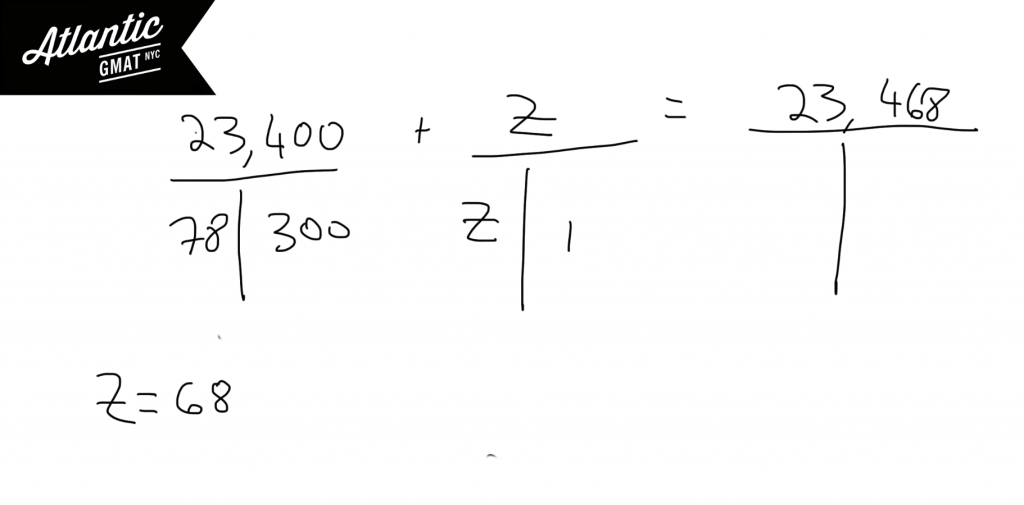# GMAT Question of the Day - Problem Solving - Average/Word Problem

For the past 300 days machine X has produced an average of 78 units of part K. Today machine X produced Z units of part K bringing machine X's total production of part K to 23,468 units. What is the value of Z?

A. 34

B. 36

C. 68

D. 72

E. 74

## GMAT Question of the Day Solution

GMAT weighted average questions are very similar to GMAT Rate/Work questions. For both question types you can use a T to organize the information. The T will help you make inferences. It can also help you to define the question. In a classic GMAT weighted average question you will have two scenarios and a total. You can organize those scenarios into three T's. Once you've done that this question of the day breaks down to simple arithmetic. Here's an Official GMAT question on which we also use the T method to organize the information: A boat traveled upstream a distance of 90 miles at an average speed of (v-3) miles per hour and then traveled the same distance downstream at an average speed of (v+3) miles per hourMore GMAT Average Rate Practice Questions:

Distance, Work and Rate - Average Rate 1

Distance, Work and Rate - Average Rate 2

For more GMAT distance, work, and rate practice visit GMAT Question of the Day.

Sorry, comments are closed for this post.

# CONTACT

Atlantic GMAT

405 East 51st St.

NY, NY 10022

(347) 669-3545

info@AtlanticGMAT.com